# Defining and Simulating Populations

##### August, 2020
using Pumas, DataFrames, LinearAlgebra, Plots


# Introduction

In this tutorial, we will cover the fundamentals of generating populations to simulate with Pumas. We will demonstrate how to specify dosage regimens and covariates, and then how to piece these together to form a population to simulate.

## The model

Below is a Pumas model that specifies a 1-compartment oral absorption system with between-subject variability on all the parameters. Details of the model specification are provided in the introduction tutorial.

model = @model begin
@param begin
θ ∈ VectorDomain(4)
Ω ∈ PSDDomain(3)
σ_prop ∈ RealDomain(init=0.1)
end

@random begin
η ~ MvNormal(Ω)
end

@covariates Wt

@pre begin
CL = θ*(Wt/70)^0.75*exp(η)
V = θ*(Wt/70)^0.75*exp(η)
Ka = θ*exp(η)
end

@dynamics begin
Depot'   = -Ka*Depot
Central' =  Ka*Depot - Central*CL/V
end

@vars begin
conc = Central/V
end

@derived begin
dv ~ @.Normal(conc,sqrt(conc^2*σ_prop+ eps()))
end

end

PumasModel
Parameters: θ, Ω, σ_prop
Random effects: η
Covariates: Wt
Dynamical variables: Depot, Central
Derived: dv, conc
Observed: dv, conc


## Setting up parameters

Next we provide the initial estimates of the parameters to simulate from. The fixed effects are provided in the θ vector (CL, V, Ka) and the between-subject variability parameteres are provided in the Ω vector as variances. So, 0.04 variance on Ω11 suggests a 20% coefficient of variation. Similarly, σprop has a 20% proportional residual error.

fixeffs = (
θ = [0.4,20,1.1,2],
Ω = diagm(0 => [0.04,0.04,0.04]),
σ_prop = 0.04
)

(θ = [0.4, 20.0, 1.1, 2.0], Ω = [0.04 0.0 0.0; 0.0 0.04 0.0; 0.0 0.0 0.04],
σ_prop = 0.04)


## Single dose example

DosageRegimen() is the function that lets you construct a dosing regimen. The first argument of the DosageRegimen is amt and is not a named argument. All subsequent arguments need to be named. Lets try a simple example where you provide a 100 mg dose at time=0.

ev = DosageRegimen(100, time=0)
first(ev.data)


DataFrameRow (10 columns)

timecmtamtevidiiaddlratedurationssroute
Float64Int64Float64Int8Float64Int64Float64Float64Int8Nothing
10.01100.010.000.00.00

As you can see above, we provided a single 100 mg dose. DosageRegimen provides some defaults when it creates the dataset, time=0, evid=1, cmt=1, rate=0, ii=0 & addl=0. We can also provide units to the amt and any other variable that is derived from amt, e.g. rate, will have associated units. Handling of units will be covered in a different tutorial.

Note that ev is of type DosageRegimen. Specified like above, DosageRegimen is one of the four fundamental building block of a Subject (more on Subject below).

### Building Subjects

Let's create a single subject

s1 = Subject(id=1,events=ev,covariates=(Wt=70,))
for fn in fieldnames(Subject)
x = getproperty(s1, fn)
if !isa(x, Nothing)
println(fn)
println(x)
end
end

id
1
covariates
Pumas.ConstantCovar{NamedTuple{(:Wt,),Tuple{Int64}}}((Wt = 70,))
events
Pumas.Event{Float64,Float64,Float64,Float64,Float64,Float64,Nothing,Int64}[
Dose event
dose amount = 100.0
dose time = 0.0
compartment = 1
instantaneous
interdose interval = 0.0
infusion start time = 0.0
]


Note that each Subject is an individual composed of:

• id: an unique identifier

• obs: observations, represented by Pumas.Observation[]

• cvs: covariates

• evs: events, represented by Pumas.Event[]

In the example above, we only provided the id, evs, and the cvs. Since obs were not provided, they are represented by an empty array. Lets take a closer at the events for this subject 1.

s1.events

1-element Array{Pumas.Event{Float64,Float64,Float64,Float64,Float64,Float64
,Nothing,Int64},1}:
Dose event
dose amount = 100.0
dose time = 0.0
compartment = 1
instantaneous
interdose interval = 0.0
infusion start time = 0.0


The events are presented by basic information such as the dose of drug and associated units if specified, the time of dose administration, the compartment number for administration and whether the dose is an instantaneous input or an infusion.

Below is how the covariates are represented

s1.covariates

Pumas.ConstantCovar{NamedTuple{(:Wt,),Tuple{Int64}}}((Wt = 70,))


(Note: defining distributions for covariates will be discussed in detail later.)

Using this one subject, s1, let us simulate a simple concentration time profile using the model above:

obs = simobs(model,s1,fixeffs,obstimes=0:0.1:120)
plot(obs)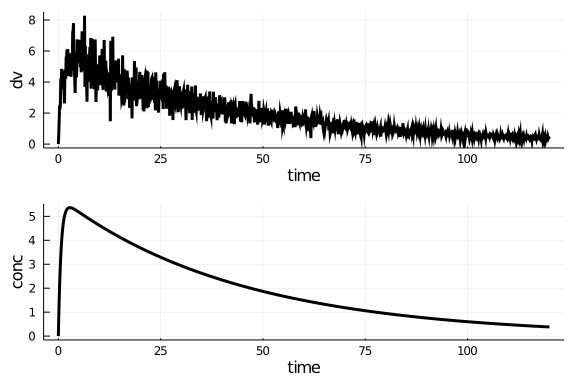### Building Populations

Now, lets create one more subject, s2.

s2 = Subject(id=2,events=ev,covariates=(Wt=70,))

Subject
ID: 2
Events: 1
Covariates: Wt


If we want to simulate both s1 and s2 together, we need to bring these subjects together to form a Population. A Population is essentially a collection of subjects.

twosubjs =  Population([s1,s2])

Population
Subjects: 2
Covariates: Wt


Let's see the details of the first and the second subject

twosubjs

Subject
ID: 1
Events: 1
Covariates: Wt

twosubjs

Subject
ID: 2
Events: 1
Covariates: Wt


Now, we can simulate this Population of 2 subjects as below

obs = simobs(model,twosubjs,fixeffs,obstimes=0:0.1:120)

2-element Array{Pumas.SimulatedObservations{Pumas.Subject{Nothing,Pumas.Con
stantCovar{NamedTuple{(:Wt,),Tuple{Int64}}},Array{Pumas.Event{Float64,Float
64,Float64,Float64,Float64,Float64,Nothing,Int64},1},Nothing},StepRangeLen{
Float64,Base.TwicePrecision{Float64},Base.TwicePrecision{Float64}},NamedTup
le{(:dv, :conc),Tuple{Array{Float64,1},Array{Float64,1}}}},1}:
Pumas.SimulatedObservations{Pumas.Subject{Nothing,Pumas.ConstantCovar{Name
dTuple{(:Wt,),Tuple{Int64}}},Array{Pumas.Event{Float64,Float64,Float64,Floa
t64,Float64,Float64,Nothing,Int64},1},Nothing},StepRangeLen{Float64,Base.Tw
icePrecision{Float64},Base.TwicePrecision{Float64}},NamedTuple{(:dv, :conc)
,Tuple{Array{Float64,1},Array{Float64,1}}}}(Subject
ID: 1
Events: 1
Covariates: Wt
, 0.0:0.1:120.0, (dv = [-2.0758854264722033e-8, 0.5849379703413957, 0.89112
01520740605, 1.2254801439315106, 1.542504268913339, 1.9618388101411581, 1.8
66426986024413, 1.8642856282353515, 2.774419560600738, 2.404760301500775  …
0.6654944001195666, 0.7236971325224688, 0.766468754533802, 0.721920353729
9739, 0.49768699041226916, 0.6421448830422739, 0.35039599055850396, 0.81813
76547825279, 0.36928000762587376, 0.42074972350915896], conc = [0.0, 0.4649
2569699238356, 0.8846399245371623, 1.2634631517963182, 1.60530122836403, 1.
9136896177402485, 2.191825262812218, 2.442595575731276, 2.6686202105431778,
2.8722655369104073  …  0.632888819492318, 0.6317960150352423, 0.6307050974
350651, 0.6296160634467626, 0.6285289098326058, 0.6274436333621599, 0.62636
02308122854, 0.6252786989671372, 0.6241990346181643, 0.6231212345641114]),
:Success)
Pumas.SimulatedObservations{Pumas.Subject{Nothing,Pumas.ConstantCovar{Name
dTuple{(:Wt,),Tuple{Int64}}},Array{Pumas.Event{Float64,Float64,Float64,Floa
t64,Float64,Float64,Nothing,Int64},1},Nothing},StepRangeLen{Float64,Base.Tw
icePrecision{Float64},Base.TwicePrecision{Float64}},NamedTuple{(:dv, :conc)
,Tuple{Array{Float64,1},Array{Float64,1}}}}(Subject
ID: 2
Events: 1
Covariates: Wt
, 0.0:0.1:120.0, (dv = [4.035860687356422e-9, 0.25854087661950365, 0.580047
2267390392, 0.6769970339013482, 1.2336733266460331, 1.2745709786893462, 1.4
234669238358764, 1.1009348556601624, 1.4049701383429114, 1.9394353035415473
…  0.9135888045511654, 0.7013125384093465, 0.726331947240408, 0.652652754
1488104, 0.8504358505785337, 0.7324544871247591, 0.6254345294307787, 0.6058
325387163638, 0.9131421875444418, 0.8328791510418672], conc = [0.0, 0.29345
93209586357, 0.5643969716557251, 0.8145100612162364, 1.045368609677171, 1.2
584225560991935, 1.4550145463135484, 1.636386554171365, 1.8036835169064775,
1.9579663262616949  …  0.7394651218274427, 0.7384224665515096, 0.737381281
646418, 0.736341565089136, 0.735303314862237, 0.7342665289539009, 0.7332312
053579126, 0.732197342073663, 0.7311649370330893, 0.7301339877076801]), :Su
ccess)


When using simobs on more than one subject, i.e., on a Population, the simulation is automatically parallelized across the subejcts.

plot(obs)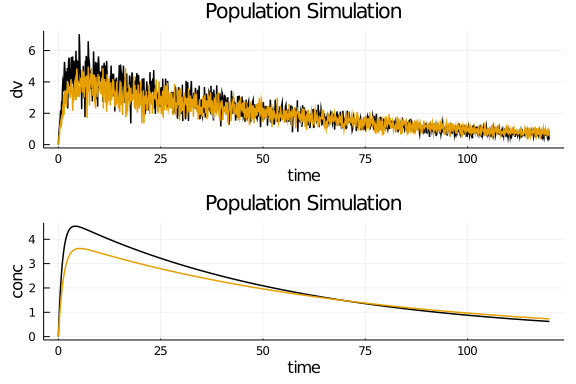Similarly, we can build a population of any number of subjects. But before we do that, let's dive into covariate generation.

### Covariates

As was discussed earlier, a Subject can also be provided details regarding covariates. In the model above, there are two covariates, isPM which stands for is the subject a poor metabolizer and takes a boolean of yes and no. The second covariate is a continuous covariate where body weight Wt impacts both CL and V. Let us now specify covariates to a population of 10 subjects.

choose_covariates() = (Wt = rand(55:80),)

choose_covariates (generic function with 1 method)


choose_covariates will randomly choose a isPM and an Wt between 55-80 kgs

We can make a list with covariates for ten subjects through a list comprehension

cvs = [ choose_covariates() for i in 1:10 ]
DataFrame(cvs)


10 rows × 1 columns

Wt
Int64
174
266
362
472
569
676
778
878
972
1065

Now, we add these covariates to the population as below. The map(f,xs) will return the result of f on each element of xs. Let's map a function that build's a subject with the randomly chosen covariates in order to build a population:

pop_with_covariates = Population(map(i -> Subject(id=i,events=ev,covariates=choose_covariates()),1:10))

Population
Subjects: 10
Covariates: Wt


Simulate into the population

obs = simobs(model,pop_with_covariates,fixeffs,obstimes=0:0.1:120);


and visualize the output

plot(obs)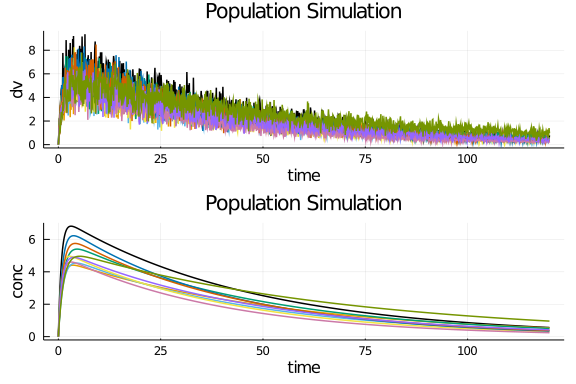## Multiple dose example

The additional dosage regimen controls of the NMTRAN format are available in DosageRegimen. For example, ii defines the "interdose interval", or the time distance between two doses, while addl defines how many additional times to repeat a dose. Thus, let's define a dose of 100 that's repeated 7 times at 24 hour intervals:

md =  DosageRegimen(100,ii=24,addl=6)


1 rows × 10 columns

timecmtamtevidiiaddlratedurationssroute
Float64Int64Float64Int8Float64Int64Float64Float64Int8Nothing
10.01100.0124.060.00.00

Let's create a new subject, s3 with this dosage regimen:

s3 = Subject(id=3,events=md, covariates=(Wt=70,))

Subject
ID: 3
Events: 7
Covariates: Wt


and see the results:

obs = simobs(model, s3, fixeffs,obstimes=0:0.1:240)
plot(obs)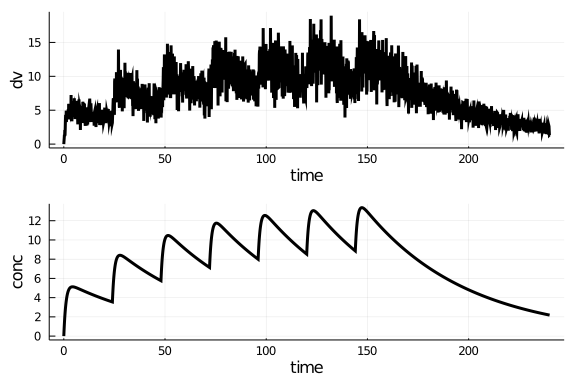## Combining dosage regimens

We can also combine dosage regimens to build a more complex regimen. Recall from the introduction that using arrays will build the element-wise combinations. Thus let's build a dose of 500 into compartment 1 at time 0, and 7 doses into compartment 1 of 100 spaced by 24 hours:

ldmd = DosageRegimen([500,100],cmt=1, time=[0,24], addl=[0,6],ii=[0,24])


2 rows × 10 columns

timecmtamtevidiiaddlratedurationssroute
Float64Int64Float64Int8Float64Int64Float64Float64Int8Nothing
10.01500.010.000.00.00
224.01100.0124.060.00.00

Let's see if this result matches our intuition:

s4 = Subject(id=4, events=ldmd, covariates=(Wt=70,))
obs = simobs(model, s4, fixeffs,obstimes=0:0.1:120)
plot(obs, ylims=(0,50))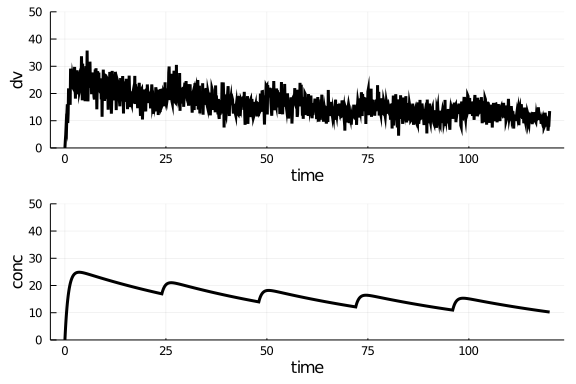Another way to build complex dosage regiments is to combine previously constructed regimens into a single regimen. For example:

e1 = DosageRegimen(500,cmt=1, time=0, addl=0,ii=0)
e2 = DosageRegimen(100,cmt=1, time=24, addl=6,ii=24)
evs = DosageRegimen(e1,e2)
obs = simobs(model, s4, fixeffs,obstimes=0:0.1:120)
plot(obs, ylims=(0,50))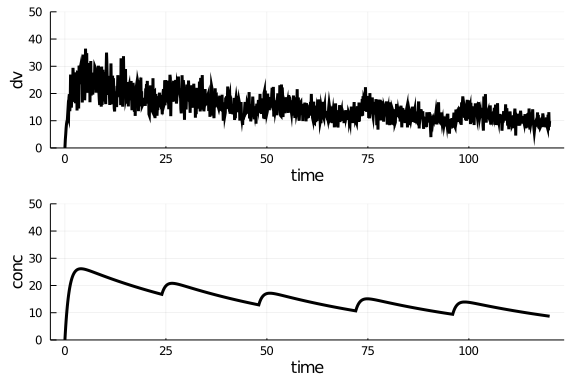is the same regimen as before.

Putting these ideas together, we can define a population where individuals with different covariates undergo different regimens, and simulate them all together with automatic parallelism:

e1 = DosageRegimen(100, ii=24, addl=6)
e2 = DosageRegimen(50,  ii=12, addl=13)
e3 = DosageRegimen(200, ii=24, addl=2)


1 rows × 10 columns

timecmtamtevidiiaddlratedurationssroute
Float64Int64Float64Int8Float64Int64Float64Float64Int8Nothing
10.01200.0124.020.00.00
pop1 = Population(map(i -> Subject(id=i,events=e1,covariates=choose_covariates()),1:5))
pop2 = Population(map(i -> Subject(id=i,events=e2,covariates=choose_covariates()),6:8))
pop3 = Population(map(i -> Subject(id=i,events=e3,covariates=choose_covariates()),9:10))
pop = Population(vcat(pop1,pop2,pop3))

Population
Subjects: 10
Covariates: Wt

obs = simobs(model,pop,fixeffs,obstimes=0:0.1:120)
plot(obs)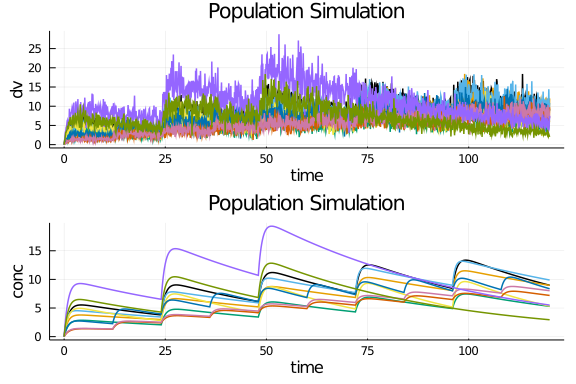## Defining Infusions

As specified in the NMTRAN format, an infusion is a dosage which is defined as having a non-zero positive rate at which the drug enters the system. Let's define a single infusion dose of total amount 100 with a rate of 3 into the second compartment:

inf = DosageRegimen(100, rate=3, cmt=2)


1 rows × 10 columns

timecmtamtevidiiaddlratedurationssroute
Float64Int64Float64Int8Float64Int64Float64Float64Int8Nothing
10.02100.010.003.033.33330

Now let's simulate a subject undergoing this treatment strategy:

s5 = Subject(id=5, events=inf, covariates=(Wt=70,))
obs = simobs(model, s5, fixeffs, obstimes=0:0.1:120)
plot(obs)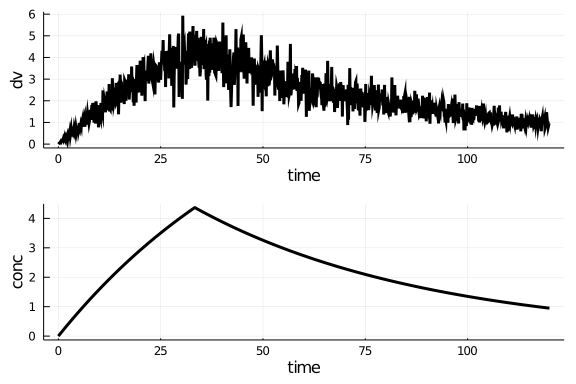## Final Note on Julia Programming

Note that all of these functions are standard Julia functions, and thus standard Julia programming constructions can be utilized to simplify the construction of large populations. We already demonstrated the use of map and a comprehension, but we can also make use of constructs like for loops.

## Conclusion

This tutorial shows the tools for generating populations of infinite complexity, defining covariates and dosage regimens on the fly and simulating the results of the model.